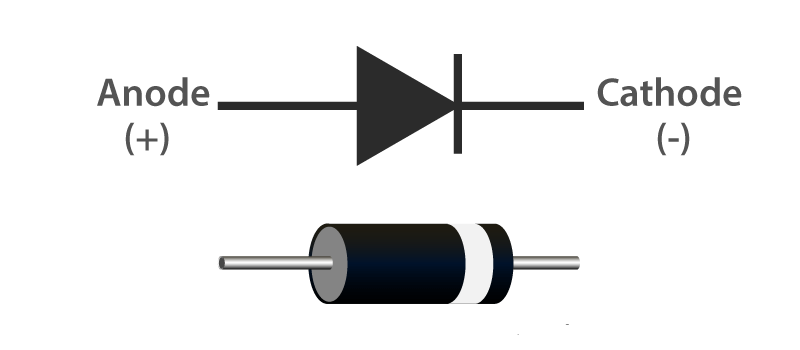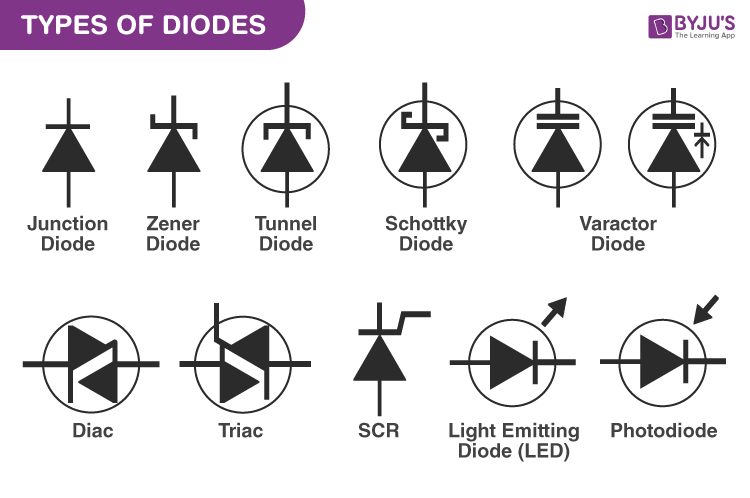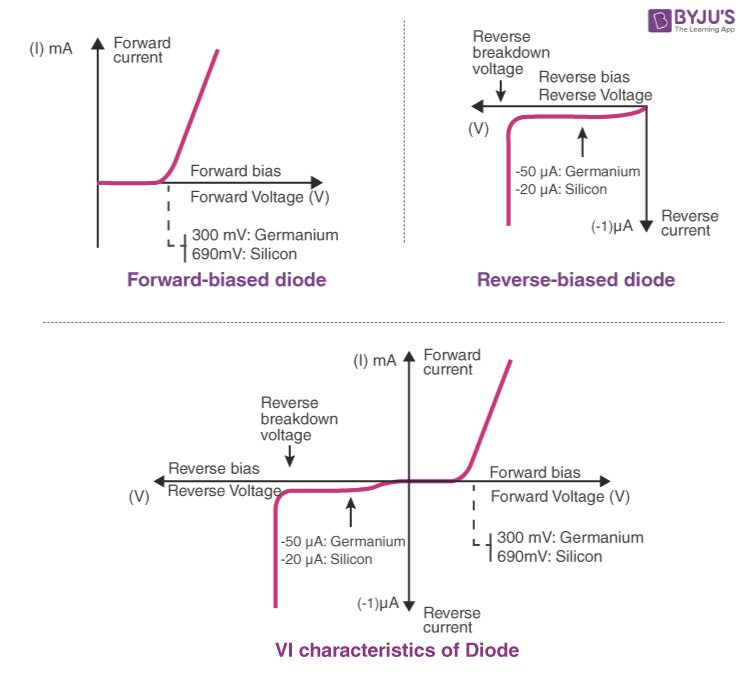# Diodes

A diode is a two-terminal electronic component that conducts electricity primarily in one direction. It has high resistance on one end and low resistance on the other end. In this article, let us understand in detail about what is diode and diode symbol.

## What is a Diode?

Diodes are used to protect circuits by limiting the voltage and to also transform AC into DC. Semiconductors like silicon and germanium are used to make the most of the diodes. Even though they transmit current in a single direction, the way with which they transmit differs. There are different kinds of diodes and each type has its own applications.

## Diode SymbolA standard diode symbol is represented as above. In the above diagram, we can see that there are two terminals that are known as anode and cathode. The arrowhead is the anode that represents the direction of the conventional current flow in the forward biased condition. The other end is the cathode.

## Diode Construction

Diodes can be made of either of the two semiconductor materials, silicon and germanium. When the anode voltage is more positive than the cathode voltage, the diode is said to be forward-biased, and it conducts readily with a relatively low-voltage drop. Likewise, when the cathode voltage is more positive than the anode, the diode is said to be reverse-biased. The arrow in the diode symbol represents the direction of conventional current flow when the diode conducts.

## Types of Diodes

1. Light Emitting Diode
2. Laser diode
3. Avalanche diode
4. Zener diode
5. Schottky diode
6. Photodiode
7. PN junction diodeDifferent Diodes and their Notation

#### Light Emitting Diode (LED)

When an electric current between the electrodes passes through this diode, light is produced. In other words, light is generated when a sufficient amount of forwarding current passes through it. In many diodes, this light generated is not visible as there are frequency levels that do not allow visibility. LEDs are available in different colours. There are tricolour LEDs that can emit three colours at a time. Light colour depends on the energy gap of the semiconductor used.

#### Laser Diode

It is a different type of diode as it produces coherent light. It is highly used in CD drives, DVDs and laser devices. These are costly when compared to LEDs and are cheaper when compared to other laser generators. Limited life is the only drawback of these diodes.

#### Avalanche Diode

This diode belongs to a reverse bias type and operates using the avalanche effect. When voltage drop is constant and is independent of current, the breakdown of avalanche takes place. They exhibit high levels of sensitivity and hence are used for photo detection.

#### Zener Diode

It is the most useful type of diode as it can provide a stable reference voltage. These are operated in reverse bias and break down on the arrival of a certain voltage. If current passing through the resistor is limited, a stable voltage is generated. Zener diodes are widely used in power supplies to provide a reference voltage.

#### Schottky Diode

It has a lower forward voltage than other silicon PN junction diodes. The drop will be seen where there is low current and at that stage, voltage ranges between 0.15 and 0.4 volts. These are constructed differently in order to obtain that performance. Schottky diodes are highly used in rectifier applications.

#### Photodiode

A photo-diode can identify even a small amount of current flow resulting from the light. These are very helpful in the detection of the light. This is a reverse bias diode and used in solar cells and photometers. They are even used to generate electricity.

#### P-N Junction Diode

The P-N junction diode is also known as rectifier diodes. These diodes are used for the rectification process and are made up of semiconductor material. The P-N junction diode includes two layers of semiconductors. One layer of the semiconductor material is doped with P-type material and the other layer with N-type material. The combination of these both P and N-type layers form a junction known as the P-N junction. Hence, the name P-N junction diode.

P-N junction diode allows the current to flow in the forward direction and blocks the flow of current in the reverse direction.

Related Articles:

## Characteristics Of Diode

The following are the characteristics of the diode:

• Forward-biased diode
• Reverse-biased diode
• Zero biased diode### Forward-biased Diode

There is a small drop of voltage across the diode when the diode is forward-biased and the current is conducting. For silicon diodes, the forward voltage is 690mV and for germanium, 300mV is the forward voltage. The potential energy across the p-type material is positive and across the n-type material, the potential energy is negative.

### Reverse-biased Diode

A diode is said to be reverse-biased when the battery’s voltage is dropped completely. For silicon diodes, the reverse voltage is -20μA and for germanium, -50μA is the reverse voltage.  The potential energy across the p-type material is negative and across the n-type material, the potential energy is positive.

### Zero-biased Diode

When the diode is zero-biased, the voltage potential across the diode is zero.

## Diode Applications

Following are the applications and uses of the diode:

• Diodes as a rectifier
• Diodes in the clipping circuit
• Diodes in clamping circuits
• Diodes in logical gates
• Diodes in reverse current protection

Hope you learned what is a diode, various types of diodes, and characteristics of diodes.

## Frequently Asked Questions – FAQs

### Can a diode be used as a rectifier?

Yes, diodes can be used as a rectifier.

### Define a diode?

A diode is a two-terminal device that has the capacity to conduct electricity in only one direction.

### Which type of semiconductor are used in manufacturing diodes?

Semiconductor materials like silicon and germanium are commonly used in diodes.

### Name the diode which has the ability to produce coherent light?

LED diodes can produce coherent light.

### Which is the diode used in solar cells and photometers?

A photodiode is used in solar cells and photometers.